# CREST Olympiads - CRO PDF Sample Papers for Class 8

Class 8 sample paper & practice questions for CREST Reasoning Olympiad (CRO) level 1 are given below. Syllabus for level 1 is also mentioned for these exams. You can refer these sample paper & quiz for preparing for the CRO exam.#### Resources:

##### Sample Questions from Olympiad Success:
 Q.1 Q.2 Q.3 Q.4 Q.5 Q.6 Q.7 Q.8 Q.9 Q.10
 Q.1 If 'red' is called 'pink', 'pink' is called 'black', 'black' is called 'white' and 'white' is called 'blue', then colour of milk is _____________. a) black b) white c) blue d) pink
 Q.2 In the following question, certain pairs of words are given, out of which the words in all pairs except one, bear a certain common relationship. Choose the odd pair. a) Tree : Stem b) Face : Eye c) Chair : Sofa d) Plant : Flower
 Q.3 Which of the following will be the correct mirror image of the given figure, when the mirror is placed vertically to the right?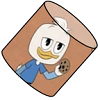a)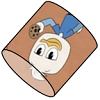b)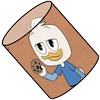c)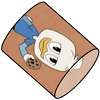d)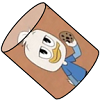Q.4 Arrange the following words as per order in the dictionary. Billian  Bifurcate  Bilateral  Bilirubin a) 2, 1, 3, 4 b) 4, 3, 2, 1 c) 2, 3, 4, 1 d) 2, 3, 1, 4
 Q.5 In the following question, four figures are given. Three are similar in a certain way and so form a group. Find out which one of the figures does not belong to that group.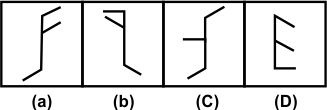a) a b) b c) c d) d
 Q.6 Which is the correct sequence of water cycle? [a] Evaporation [b] Rain [c] Sea [d] Clouds a) a, b, c, d b) d, a, b, c c) c, a, d, b d) b, d, a, c
 Q.7 Seema is the daughter of Rashmi. Mohit is the brother of Rashmi. Swati is the wife of Mohit. How is Swati related to Seema? a) Sister b) Aunt c) Mother d) Grandmother
 Q.8 Count the number of small cubes in the following figure.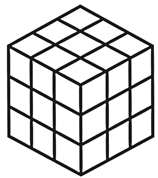a) 36 b) 32 c) 30 d) 27
 Q.9 In the following question, choose the correct mirror image of the given figure (X), if a mirror is placed vertically on the left side of the figure.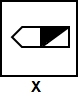a)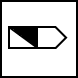b)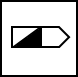c)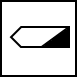d)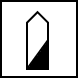Q.10 Rajesh is facing west. He turns 45° in the clockwise direction and then another 180° in the same direction and then 270° in the anticlockwise direction. Which direction is he facing now? a) South-West b) North-West c) West d) SouthSample PDF of CREST Olympiads - CREST Reasoning Olympiad (CRO) PDF Sample Papers for Class 8:

 Q.1 )c Q.2 )c Q.3 )b Q.4 )c Q.5 )d Q.6 )c Q.7 )b Q.8 )a Q.9 )a Q.10 )a

Q.1 : c | Q.2 : c | Q.3 : b | Q.4 : c | Q.5 : d | Q.6 : c | Q.7 : b | Q.8 : a | Q.9 : a | Q.10 : a